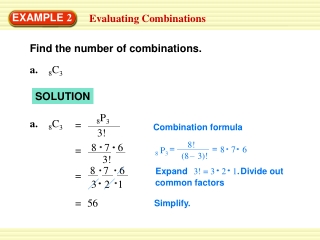Download PresentationEXAMPLE 2

# EXAMPLE 2 - PowerPoint PPT PresentationDownload Presentation## EXAMPLE 2

- - - - - - - - - - - - - - - - - - - - - - - - - - - E N D - - - - - - - - - - - - - - - - - - - - - - - - - - -
##### Presentation Transcript

1. a. 8C3 8P3 a. 8C3 = 3! 8! 8 7 6 = = = 8 7 6 8 P3 – (8 3)! 3! 8 7 6 = Expand 3! = 3 2 1. Divide out 3 2 1 common factors = 56 EXAMPLE 2 Evaluating Combinations Find the number of combinations. SOLUTION Combination formula Simplify.

2. 9P7 = 7! 9 P7 9! 9 8 7 6 5 4 3 = = – (9 7)! 7! 9 8 7 6 5 4 3 = 4 9 8 7 6 5 4 3 = 7 6 5 4 3 2 1 1 36 = EXAMPLE 2 Evaluating Combinations b. 9C 7 Combination formula Expand 7!. Divide out common factors. Simplify.

3. ANSWER 8C8 = = 1 for Example 2 GUIDED PRACTICE Find the number of combinations. 2.8C8

4. ANSWER 8C7 = = 8 for Example 2 GUIDED PRACTICE 3.8C7

5. ANSWER 7C2 = = 21 for Example 2 GUIDED PRACTICE 4.7C2

6. ANSWER 6C1 = 6 = for Example 2 GUIDED PRACTICE 5.6C1

7. Bookstore 6. You are shopping at a bookstore. You have enough money to buy 4books. There are 7 books at the store that you want to read. How many combinations of books can be purchased? ANSWER There are 35 combinations of books can be purchased. for Example 2 GUIDED PRACTICE